Method

Elbrus GPU Visual SLAM [st] [IsaacElbrusGPUSLAM]

Submitted on 15 Oct. 2021 10:41 by
Dmitry Slepichev (NVIDIA)

 Running time: 0.007 s Environment: Jetson AGX

 Method Description: Elbrus is a Stereo SLAM technology. It assumes a calibrated stereo rig (intrinsics and extrinsic of the cameras are known, including the baseline distance) and known lens distortion parameters (Brown's distortion model). Elbrus contains two major components. The 2D component is based on multi-pyramid KLT feature tracking with local average luminance compensation. The 3D component uses keyframes selected using 2D track average motion and rate of disappearance criteria. The 2D tracking of each feature is guided by the prediction of the track's position provided by the results of 3D tracking. 3D tracking is done via the exponential mapping formalism for recovering the six absolute orientation parameters of the camera rig using standard elements of triangulation, resectioning and sparse bundle adjustment (SBA). SBA runs on a separate thread in parallel with the rest of the Elbrus VO calculations. For NVIDIA Xavier Elbrus can track on 144fps. Parameters: -lr_tracker=lk_horizontal -use_gpu=true Latex Bibtex: @unpublished{ELBRUS2018, title={Realtime Stereo Visual Odometry}, author={ Alexander Korovko and Dmitry Robustov and Dmitry Slepichev and Eugene Vendrovsky and Stanislav Volodarskiy }, }

Detailed Results

From all test sequences (sequences 11-21), our benchmark computes translational and rotational errors for all possible subsequences of length (5,10,50,100,150,...,400) meters. Our evaluation ranks methods according to the average of those values, where errors are measured in percent (for translation) and in degrees per meter (for rotation). Details for different trajectory lengths and driving speeds can be found in the plots underneath. Furthermore, the first 5 test trajectories and error plots are shown below.

Test Set Average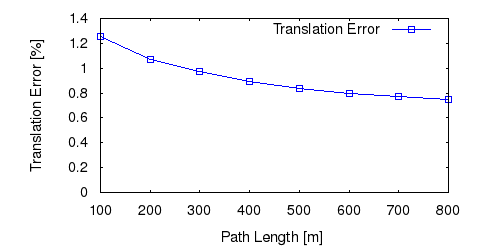This figure as: png eps pdf txt gnuplot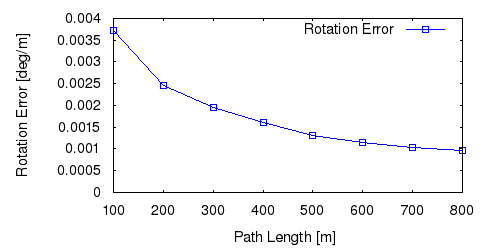This figure as: png eps pdf txt gnuplotThis figure as: png eps pdf txt gnuplot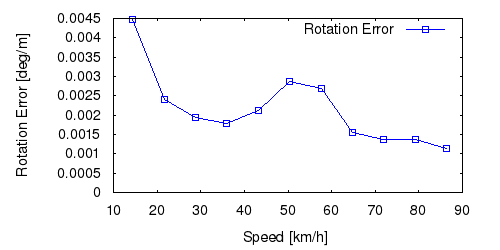This figure as: png eps pdf txt gnuplot

Sequence 11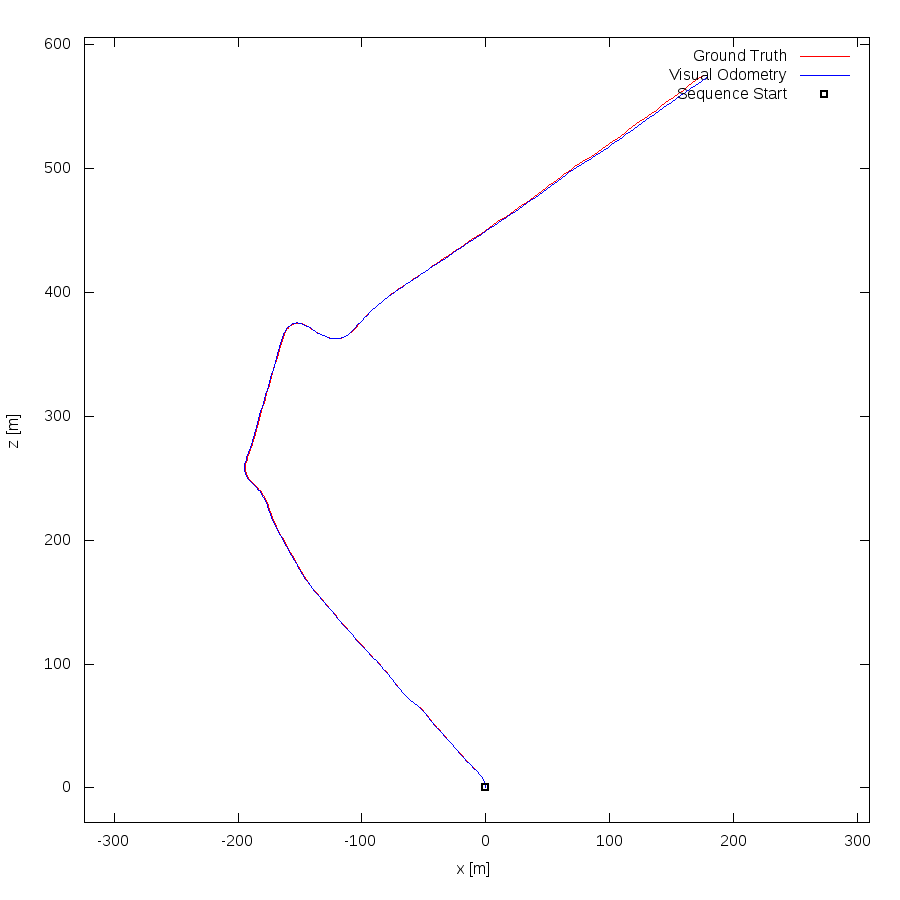This figure as: png eps pdf txt gnuplot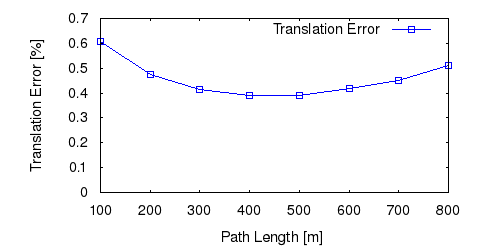This figure as: png eps pdf txt gnuplotThis figure as: png eps pdf txt gnuplotThis figure as: png eps pdf txt gnuplotThis figure as: png eps pdf txt gnuplot

Sequence 12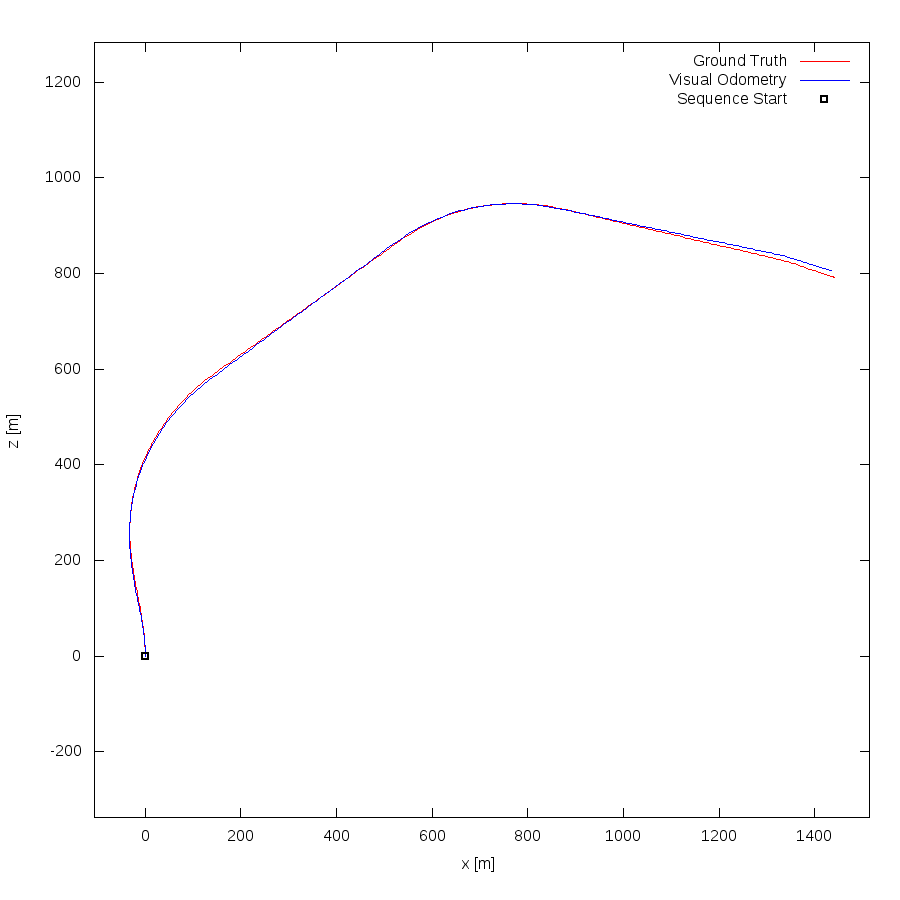This figure as: png eps pdf txt gnuplot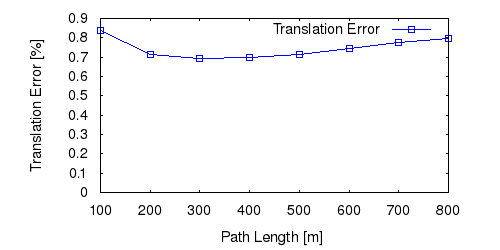This figure as: png eps pdf txt gnuplotThis figure as: png eps pdf txt gnuplot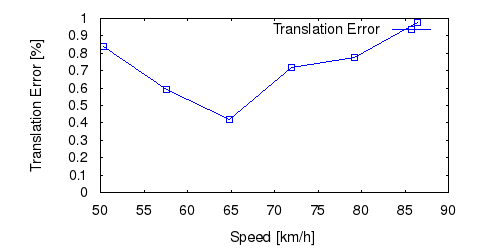This figure as: png eps pdf txt gnuplot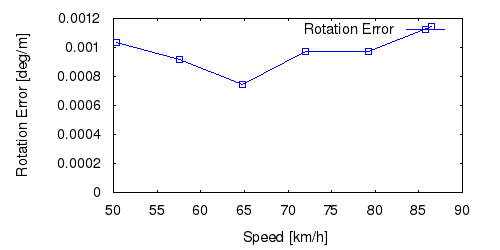This figure as: png eps pdf txt gnuplot

Sequence 13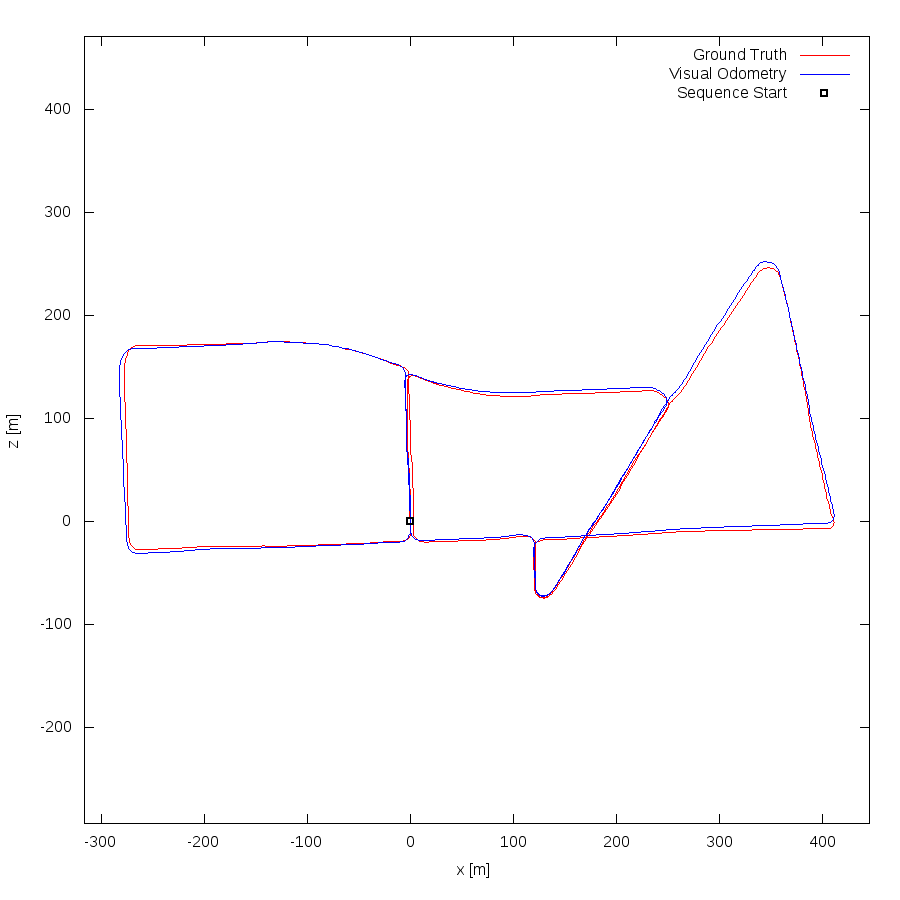This figure as: png eps pdf txt gnuplot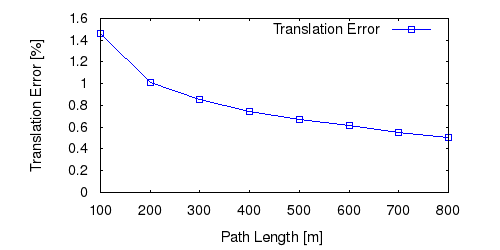This figure as: png eps pdf txt gnuplot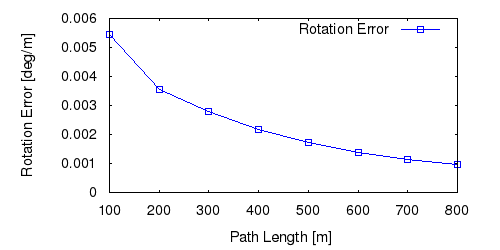This figure as: png eps pdf txt gnuplot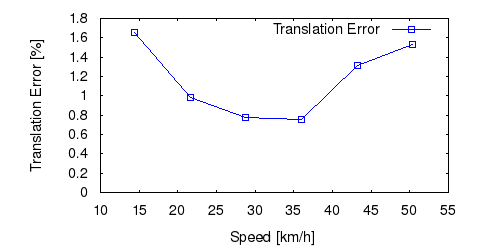This figure as: png eps pdf txt gnuplot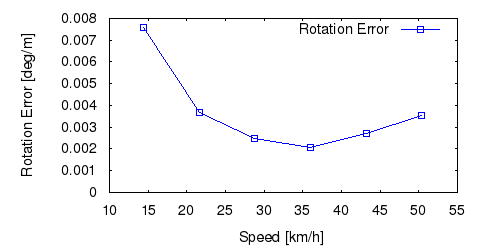This figure as: png eps pdf txt gnuplot

Sequence 14This figure as: png eps pdf txt gnuplot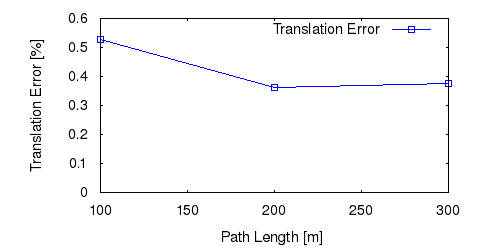This figure as: png eps pdf txt gnuplotThis figure as: png eps pdf txt gnuplot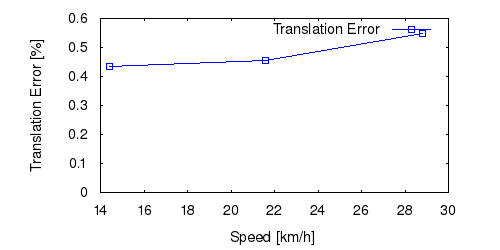This figure as: png eps pdf txt gnuplot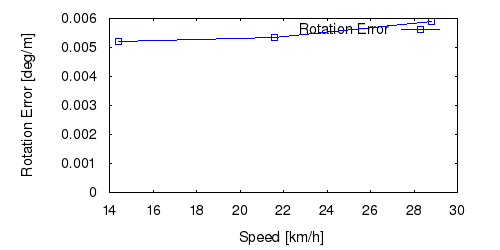This figure as: png eps pdf txt gnuplot

Sequence 15This figure as: png eps pdf txt gnuplotThis figure as: png eps pdf txt gnuplot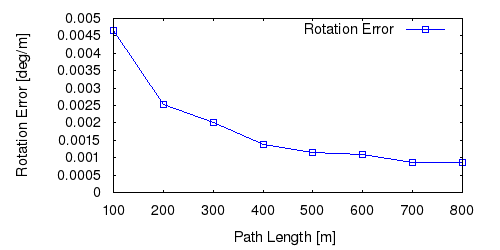This figure as: png eps pdf txt gnuplot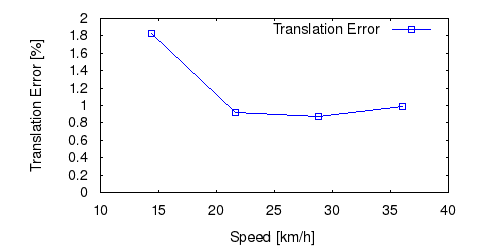This figure as: png eps pdf txt gnuplotThis figure as: png eps pdf txt gnuplot